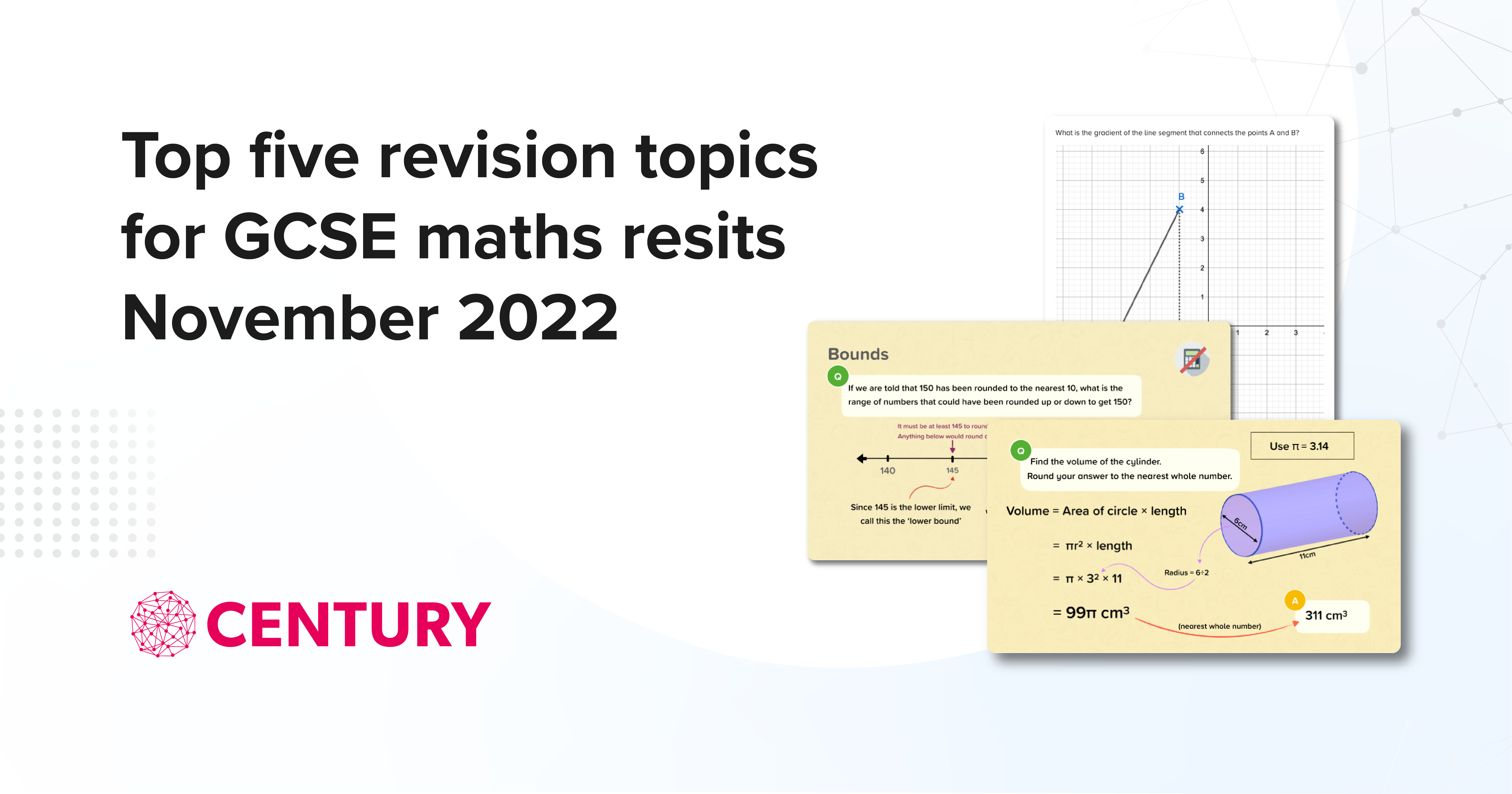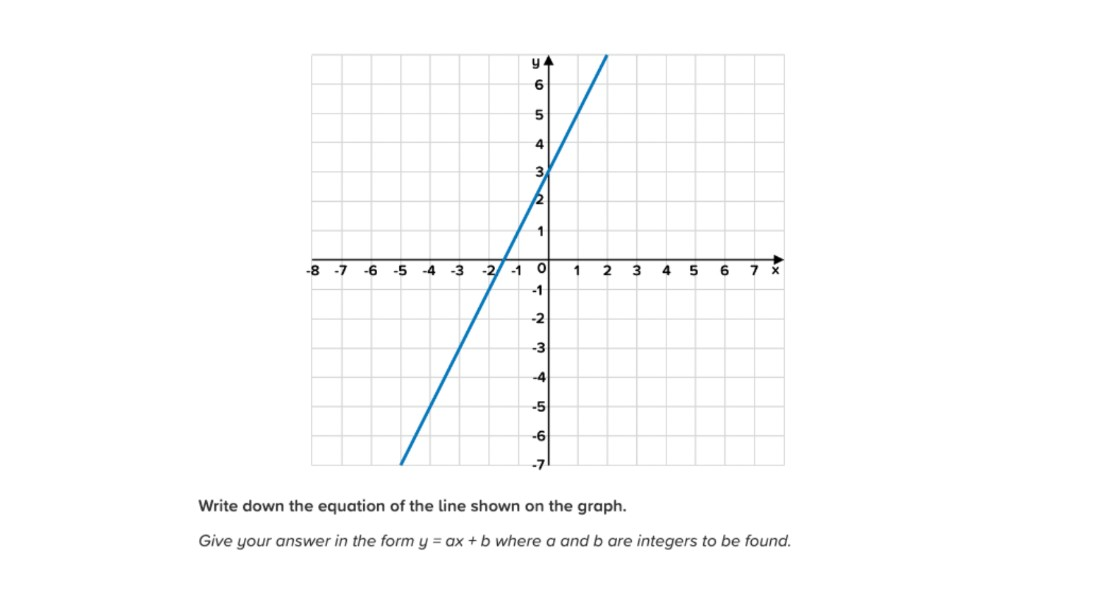# CENTURY’s top five recommended revision topics for GCSE maths resits November 2022

Posted on 3rd October 2022

Posted by CENTURY Maths Team

Share:The topics below were all in our Advance Information course in the summer term, and these are the questions that students struggled with the most. All of these topics are due to come up again in November in either the Edexcel or AQA papers, or both. We recommend you make these a priority with your students.

You can see the full information from the exam boards on the November exams Advance Information here for Edexcel and here for AQA.

The Edexcel examiner report for the June 2022 Foundation papers also indicates that the questions on these topics were poorly answered by many candidates. The misconceptions identified below are similar for the cohort wide feedback. Read the full examiner reports here.

For each of these topics we will provide a full breakdown including common incorrect answers and misconceptions we have identified from the answers students submitted. This is 3 out of 5 in our series of topics. Topics 4 and 5 will be published in the following weeks.Some ideas to build these topics into your teaching

• Set the recommended nuggets as independent learning using the planner tool on CENTURY
• Include these topics in your lessons as a starter each lesson
• Set a mini assessment for students including these topics using past exam paper questions

# Equation of a Straight Line Graph in the Form y = mx + c

This calculator question was answered incorrectly 88 percent of the time. The question required students to have knowledge of equations of straight lines in the form y = mx + c. Students need to recognise the y-intercept and be able to calculate the gradient of a straight line.Some of the most common incorrect answers were:

• 3 - Students inputting this as an answer clearly know that 3 is an important part of this graph, but do not have the understanding of how to construct an equation.
• Answers involving 3 and –1.5 - as before students know that 3 forms part of the equation but do not have knowledge of how to calculate the gradient, instead finding where the graph crosses the x axis.
• There were a variety of answers in equation form involving the coefficients 2 and 3, however not correct, eg y = –2x + 3, y = 2 + 3

This is a skill that can be built up in stages to help gain confidence. For a series of graphs the equation could be written y = 3x + __ (for example) with students needing to use their knowledge to fill in the y intercept. Then some scaffolded questions on finding the gradient, then filling the blank of y = _x + 2. Students should be familiar with drawing a triangle connecting 2 points to count the squares and calculate gradient using rise over run, or similar. They should also recognise by inspection a positive or negative gradient.

To help your students with this topic use CENTURY nuggets: Finding the Gradient of a Line Segment: Using the Graph (MF23.08), Understanding y = mx + c (MF23.10) and Graphing y = mx + c (1) (MF23.11)

Book a demo to find out more about the courses, or speak to your Account Manager if you are an existing customer for more information.